# Chapter 11 Chemical Reactions 1 Chemical Reactions o

• Slides: 44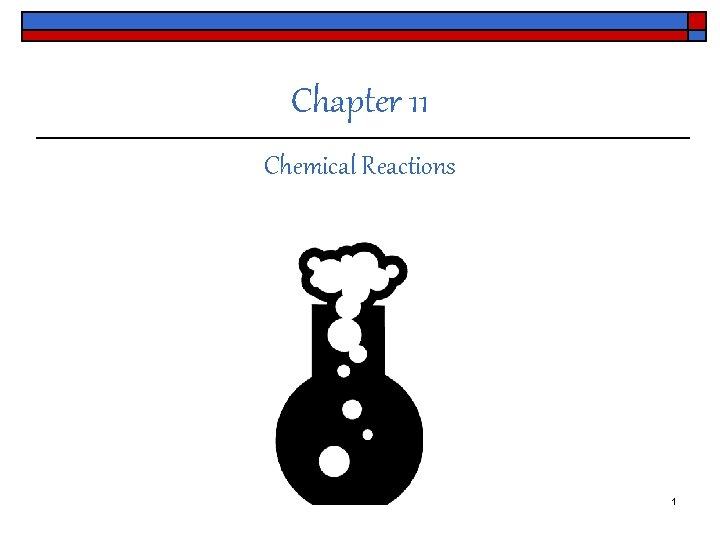Chapter 11 Chemical Reactions 1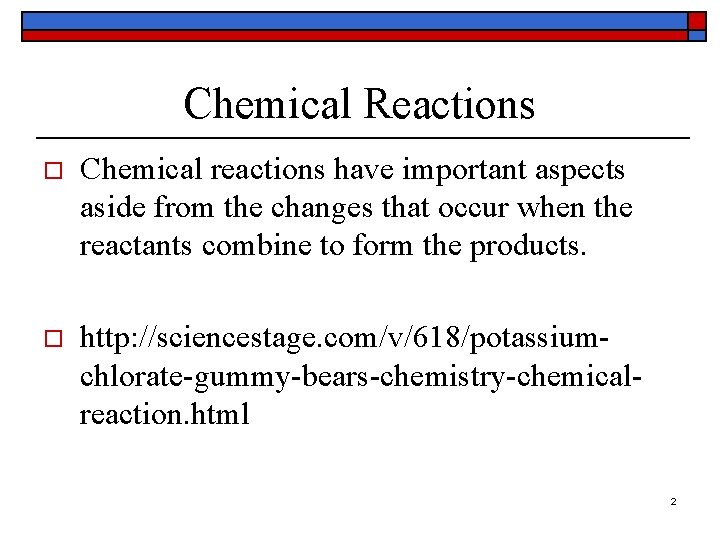Chemical Reactions o Chemical reactions have important aspects aside from the changes that occur when the reactants combine to form the products. o http: //sciencestage. com/v/618/potassiumchlorate-gummy-bears-chemistry-chemicalreaction. html 2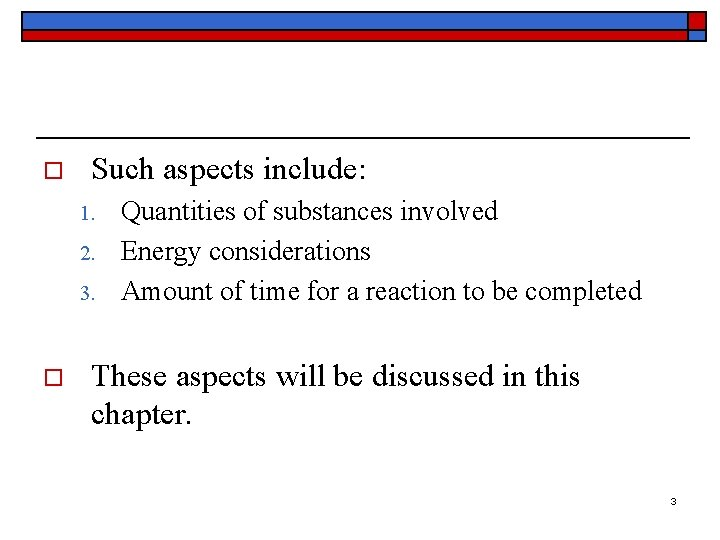o Such aspects include: 1. 2. 3. o Quantities of substances involved Energy considerations Amount of time for a reaction to be completed These aspects will be discussed in this chapter. 3History o The most spectacular chemical change our ancestors were familiar with was combustion – the process of burning. 4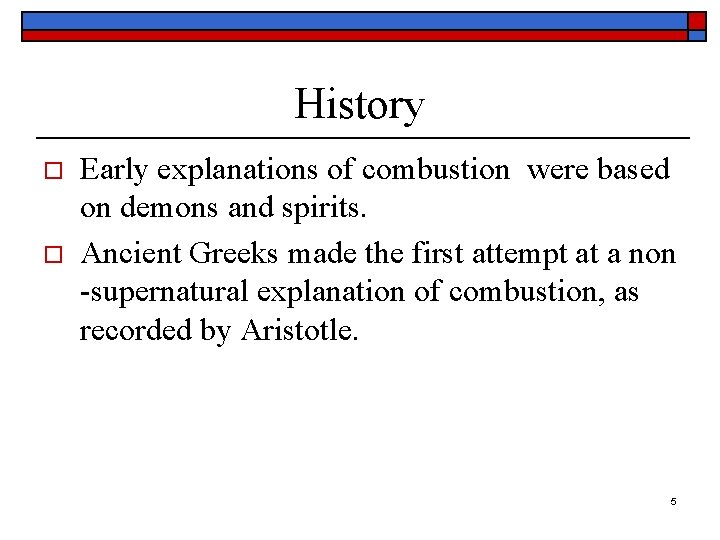History o o Early explanations of combustion were based on demons and spirits. Ancient Greeks made the first attempt at a non -supernatural explanation of combustion, as recorded by Aristotle. 5History Fire as a basic substance: o • • • Developed by Becher (1635 -1682) and Stahl (1660 -1734) into the phlogiston hypothesis. Phlogiston was defined as a SUBSTANCE that supposedly escaped during combustion. All substances that can be burned were supposed to contain phlogiston, which escapes as the burning takes place. 6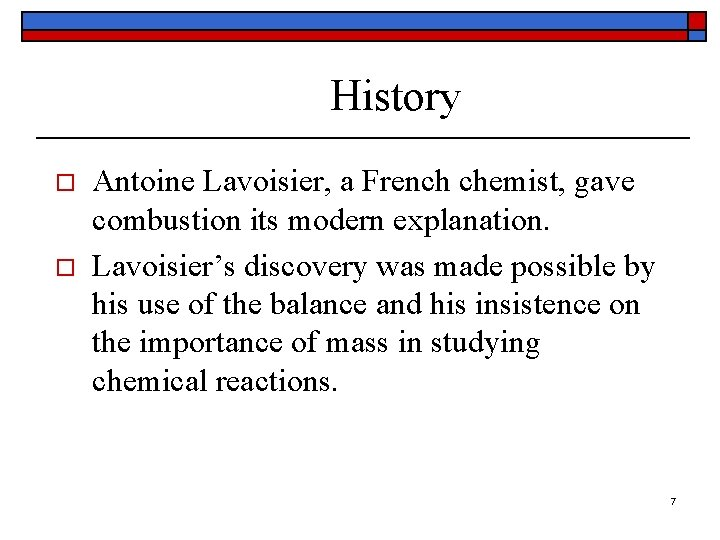History o o Antoine Lavoisier, a French chemist, gave combustion its modern explanation. Lavoisier’s discovery was made possible by his use of the balance and his insistence on the importance of mass in studying chemical reactions. 7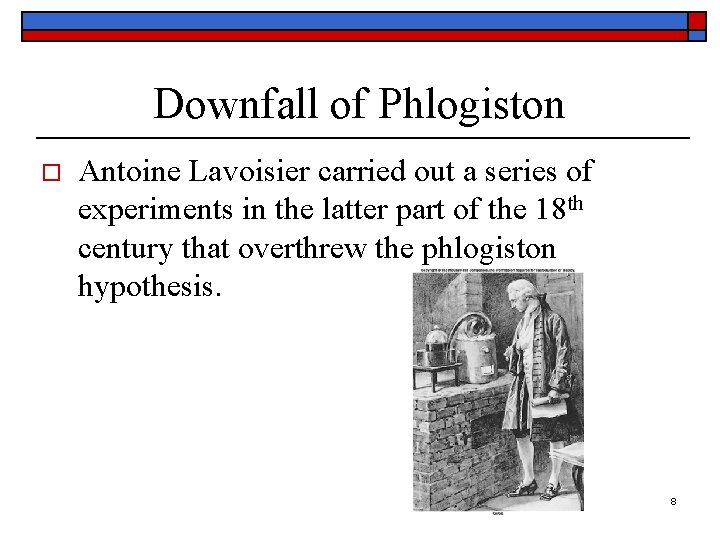Downfall of Phlogiston o Antoine Lavoisier carried out a series of experiments in the latter part of the 18 th century that overthrew the phlogiston hypothesis. 8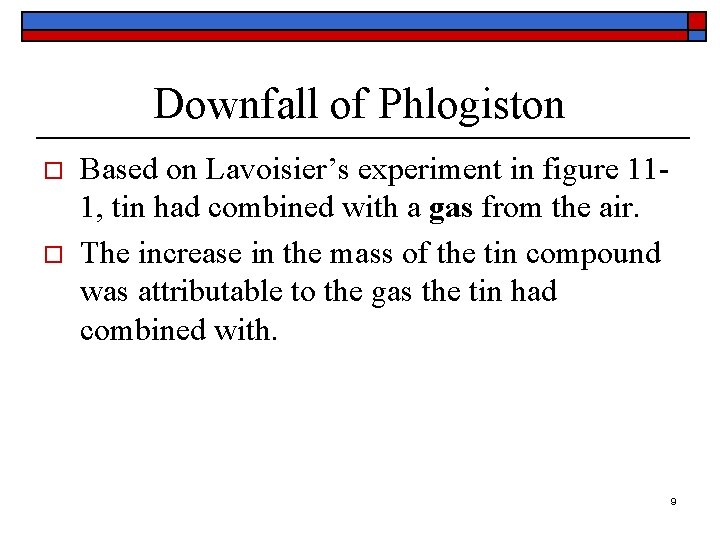Downfall of Phlogiston o o Based on Lavoisier’s experiment in figure 111, tin had combined with a gas from the air. The increase in the mass of the tin compound was attributable to the gas the tin had combined with. 9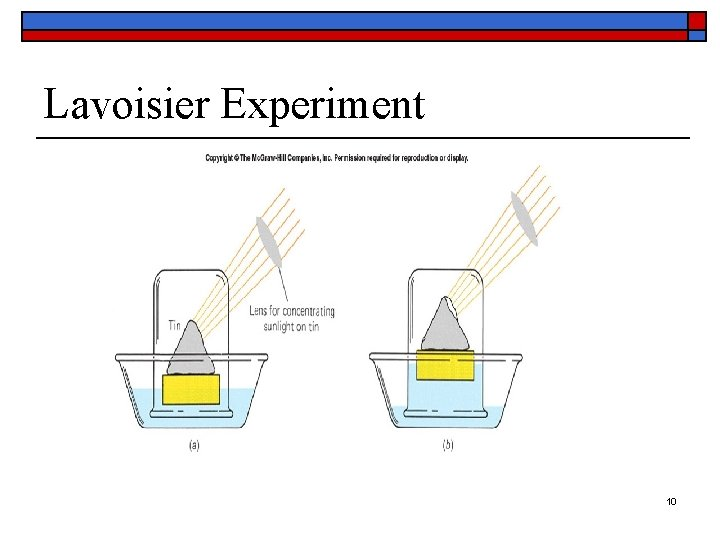Lavoisier Experiment 10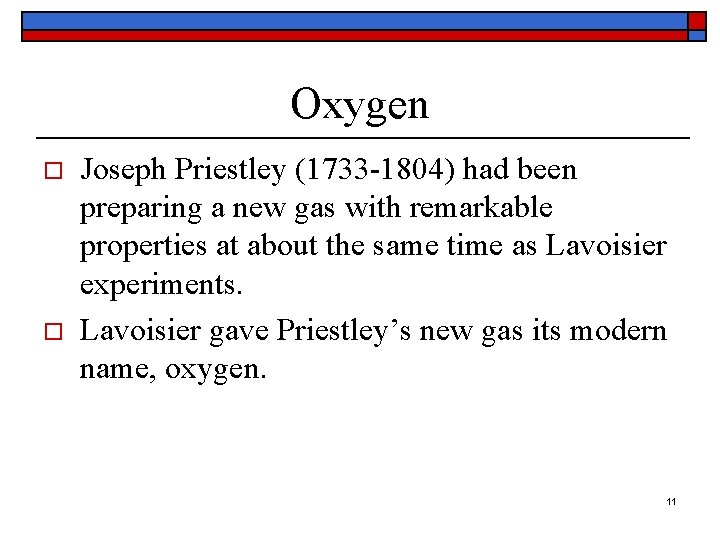Oxygen o o Joseph Priestley (1733 -1804) had been preparing a new gas with remarkable properties at about the same time as Lavoisier experiments. Lavoisier gave Priestley’s new gas its modern name, oxygen. 11Combustion o o o Lavoisier found oxygen to be involved not only in changes that occur in metals when heated but in the process of combustion as well Combustion is the process of burning Combustion is rapid oxidation 12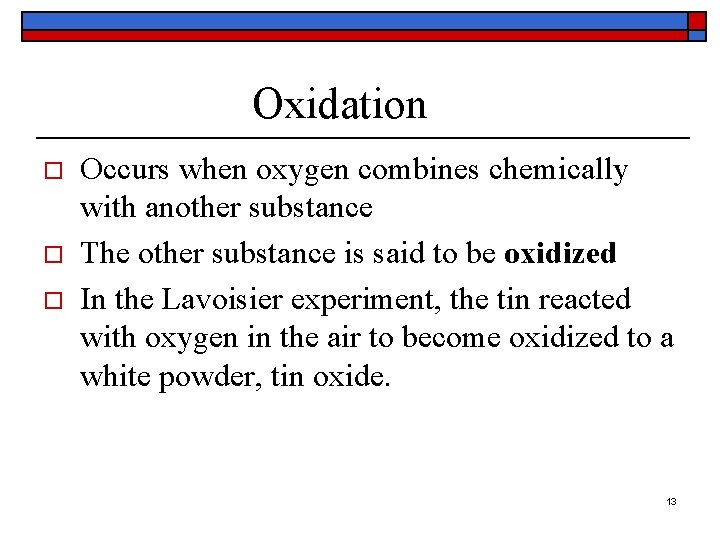Oxidation o o o Occurs when oxygen combines chemically with another substance The other substance is said to be oxidized In the Lavoisier experiment, the tin reacted with oxygen in the air to become oxidized to a white powder, tin oxide. 13Rapid and Slow Oxidation o o Rapid oxidation in which a lot of heat and light are given off is the process of combustion Slow oxidation examples include rusting of iron and the steady oxidation of food by oxygen in our bodies 14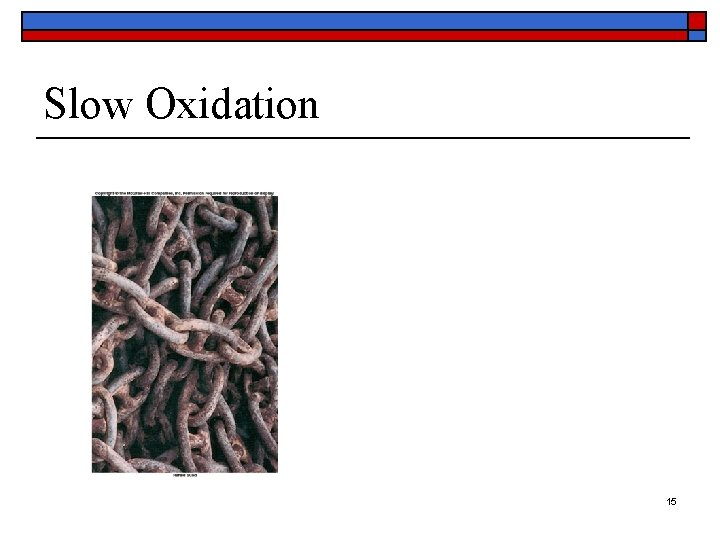Slow Oxidation 15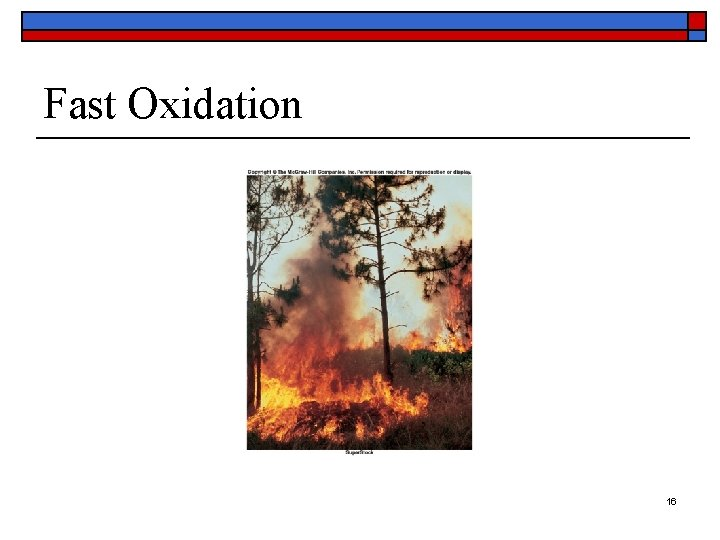Fast Oxidation 16Oxides o A substance formed by the union of another element with oxygen is called an oxide. n n o o o Tin oxide Iron oxide (rust) Carbon dioxide Silicon dioxide Generally, oxides of metals are solids Oxides of other elements may be solid, liquid or gas Oxides of nearly all the elements can be prepared, most of them simply by heating the elements with oxygen 17What is a mole? o o The chemist needs a way to relate the number of atoms in a chemical formula or equation to the corresponding masses of the substances A mole (abbreviated mol) of any element is that amount of it whose mass in grams is equal to its atomic mass expressed in u. 18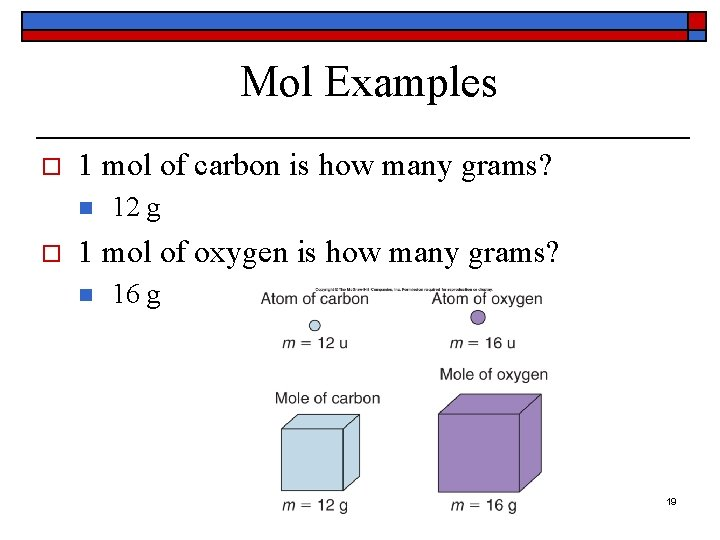Mol Examples o 1 mol of carbon is how many grams? n o 12 g 1 mol of oxygen is how many grams? n 16 g 19Avogadro’s Number (N 0) o o The number of atoms per mol of any element N 0 = 6. 02 X 1023 atoms/mol Avogadro’s number is a constant A mol of any element contains 6. 02 X 1023 atoms o o See figure 11 -7 The number of atoms in a sample of any element is the number of moles in the sample multiplied by N 0 20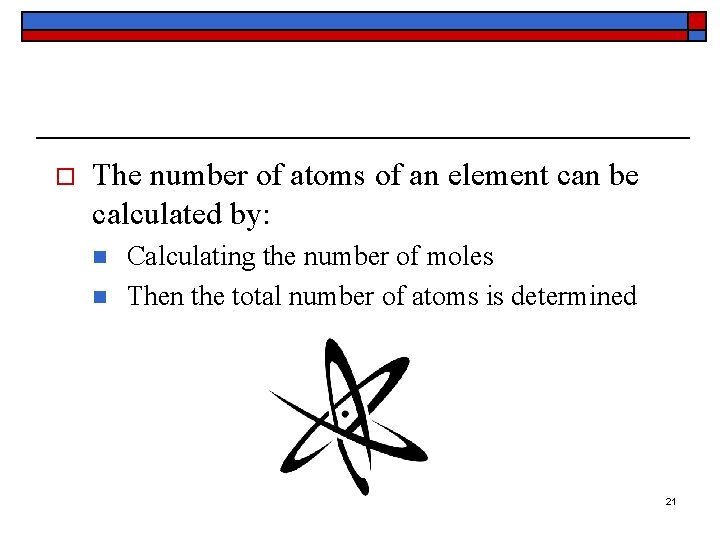o The number of atoms of an element can be calculated by: n n Calculating the number of moles Then the total number of atoms is determined 21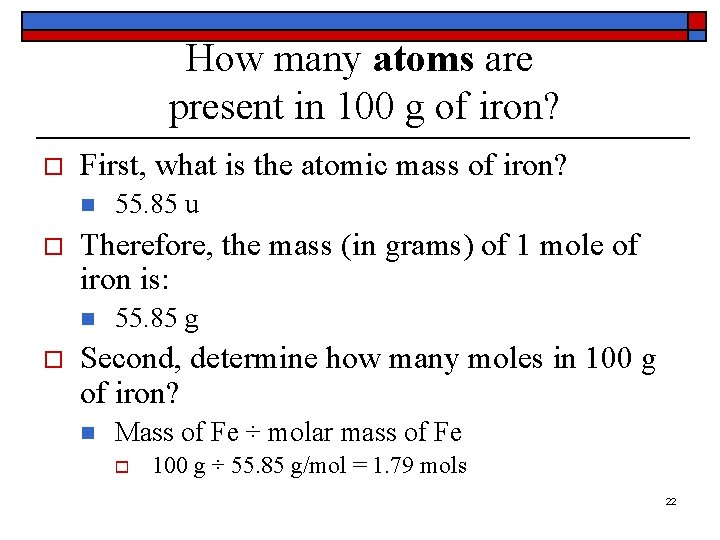How many atoms are present in 100 g of iron? o First, what is the atomic mass of iron? n o Therefore, the mass (in grams) of 1 mole of iron is: n o 55. 85 u 55. 85 g Second, determine how many moles in 100 g of iron? n Mass of Fe ÷ molar mass of Fe o 100 g ÷ 55. 85 g/mol = 1. 79 mols 22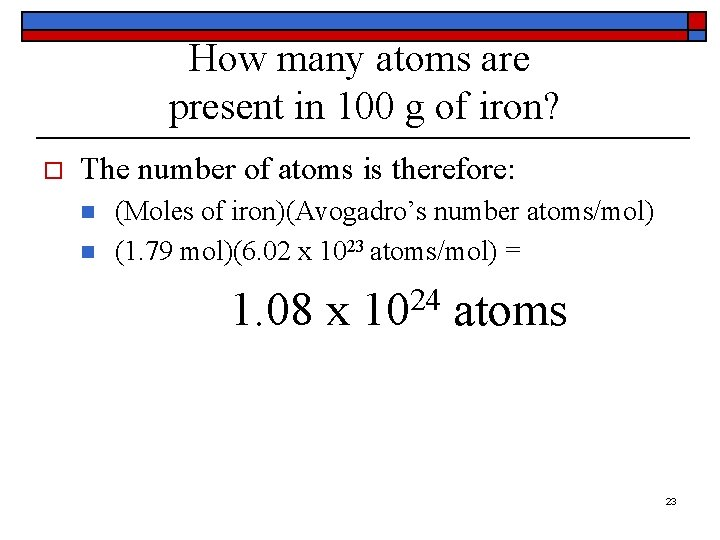How many atoms are present in 100 g of iron? o The number of atoms is therefore: n n (Moles of iron)(Avogadro’s number atoms/mol) (1. 79 mol)(6. 02 x 1023 atoms/mol) = 24 1. 08 x 10 atoms 23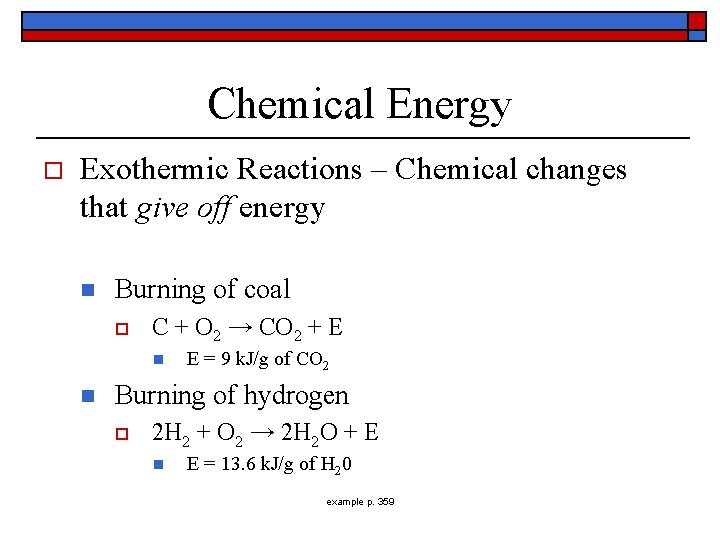Chemical Energy o Exothermic Reactions – Chemical changes that give off energy n Burning of coal o C + O 2 → CO 2 + E n n E = 9 k. J/g of CO 2 Burning of hydrogen o 2 H 2 + O 2 → 2 H 2 O + E n E = 13. 6 k. J/g of H 20 example p. 359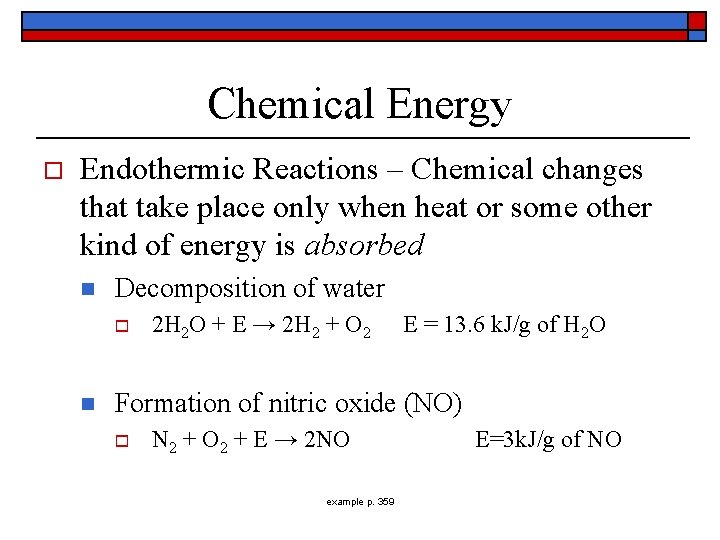Chemical Energy o Endothermic Reactions – Chemical changes that take place only when heat or some other kind of energy is absorbed n Decomposition of water o n 2 H 2 O + E → 2 H 2 + O 2 E = 13. 6 k. J/g of H 2 O Formation of nitric oxide (NO) o N 2 + O 2 + E → 2 NO example p. 359 E=3 k. J/g of NO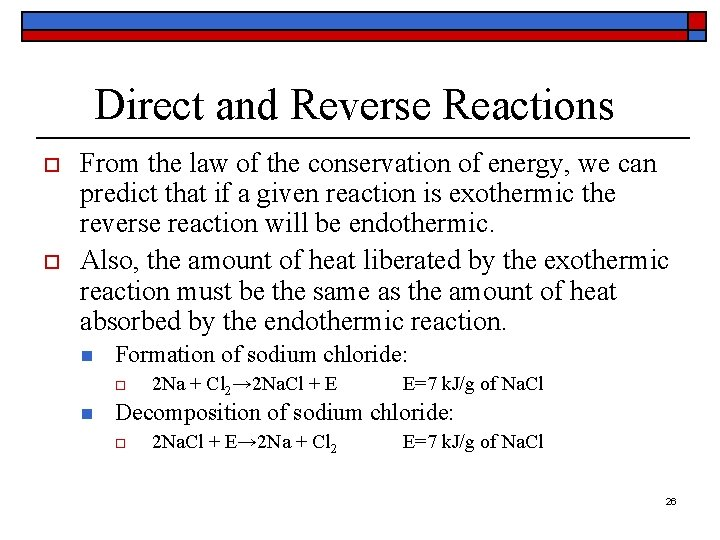Direct and Reverse Reactions o o From the law of the conservation of energy, we can predict that if a given reaction is exothermic the reverse reaction will be endothermic. Also, the amount of heat liberated by the exothermic reaction must be the same as the amount of heat absorbed by the endothermic reaction. n Formation of sodium chloride: o n 2 Na + Cl 2→ 2 Na. Cl + E E=7 k. J/g of Na. Cl Decomposition of sodium chloride: o 2 Na. Cl + E→ 2 Na + Cl 2 E=7 k. J/g of Na. Cl 26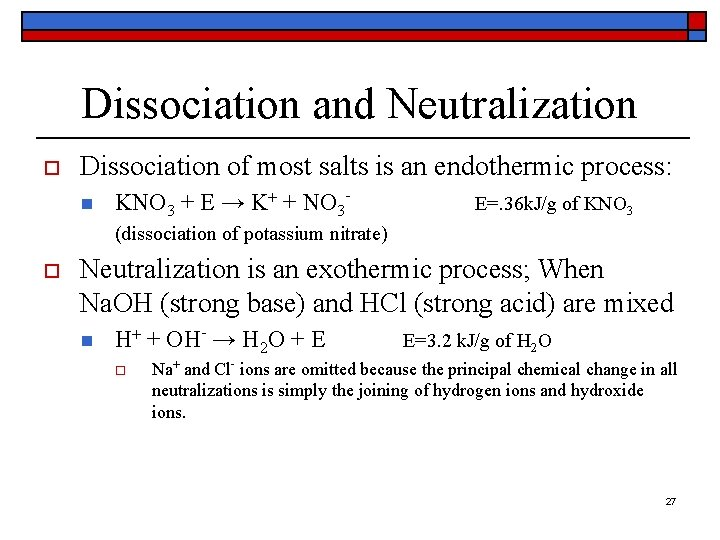Dissociation and Neutralization o Dissociation of most salts is an endothermic process: n KNO 3 + E → K+ + NO 3 - E=. 36 k. J/g of KNO 3 (dissociation of potassium nitrate) o Neutralization is an exothermic process; When Na. OH (strong base) and HCl (strong acid) are mixed n H+ + OH- → H 2 O + E o E=3. 2 k. J/g of H 2 O Na+ and Cl- ions are omitted because the principal chemical change in all neutralizations is simply the joining of hydrogen ions and hydroxide ions. 27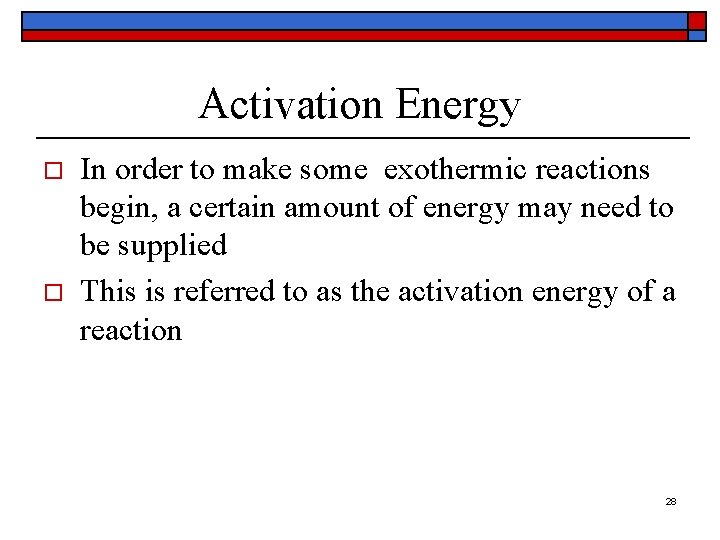Activation Energy o o In order to make some exothermic reactions begin, a certain amount of energy may need to be supplied This is referred to as the activation energy of a reaction 28Activation Energy 29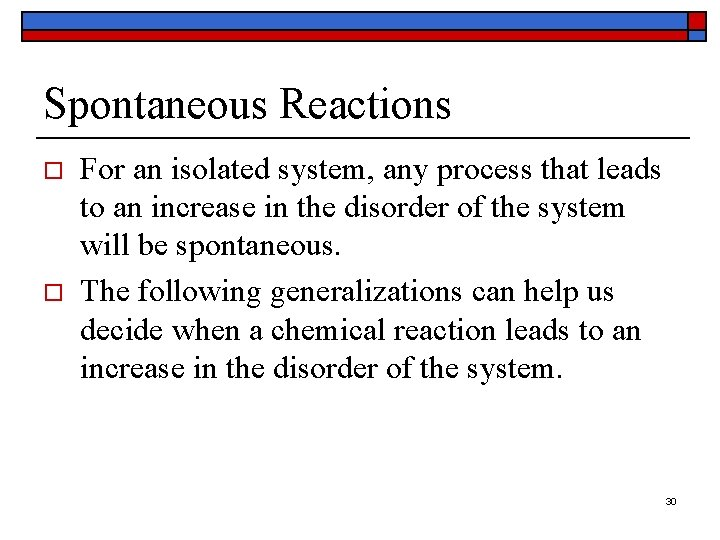Spontaneous Reactions o o For an isolated system, any process that leads to an increase in the disorder of the system will be spontaneous. The following generalizations can help us decide when a chemical reaction leads to an increase in the disorder of the system. 30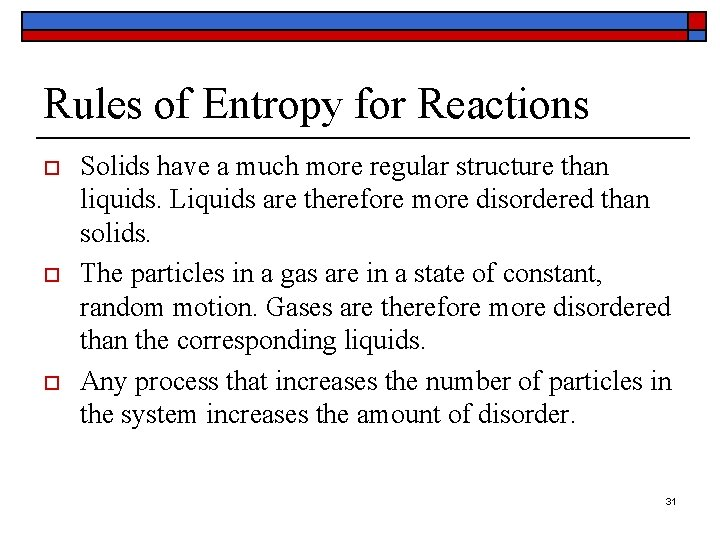Rules of Entropy for Reactions o o o Solids have a much more regular structure than liquids. Liquids are therefore more disordered than solids. The particles in a gas are in a state of constant, random motion. Gases are therefore more disordered than the corresponding liquids. Any process that increases the number of particles in the system increases the amount of disorder. 31Which of the following processes will lead to an increase in the entropy of the system? o o (a) N 2(g) + 3 H 2 (g) 2 NH 3(g) (b) H 2 O(l) H 2 O(g) (c) Ca. CO 3(s) Ca. O(s) + CO 2(g) (d) NH 4 NO 3(s) + H 2 O(l) NH 4+ (aq) + NO 3 - (aq) 32Answers to Entropy Questions o o (a) Because the total number of molecules decreases in this reaction, the system becomes more ordered. The entropy of the system therefore decreases. (b) Gases are more disordered than the corresponding liquids, so the entropy of the system increases. (c) Reactions in which a compound decomposes into two products lead to an increase in entropy because the system becomes more disordered. The increase in entropy in this reaction is even larger because the starting material is a solid and one of the products is a gas. (d) The NH 4+ and NO 3 - ions are free to move in a random fashion through the aqueous solution, whereas these ions are locked into position in the crystal. As a result, the entropy of the system increases in this reaction. 33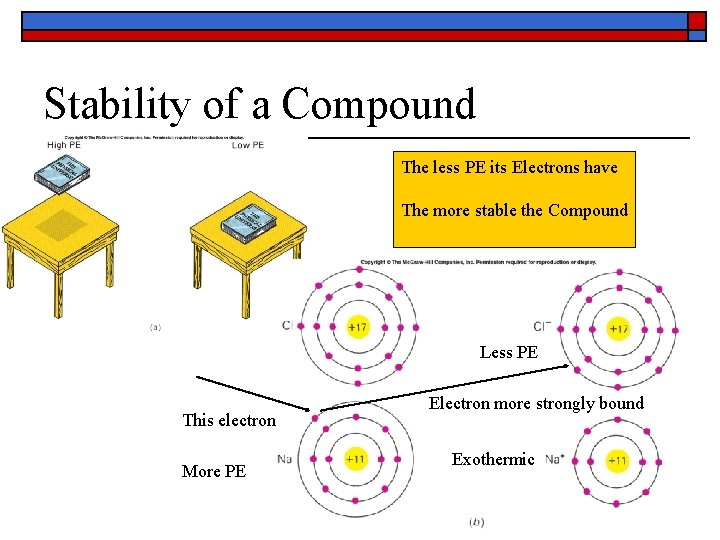Stability of a Compound The less PE its Electrons have The more stable the Compound Less PE This electron More PE Electron more strongly bound Exothermic 34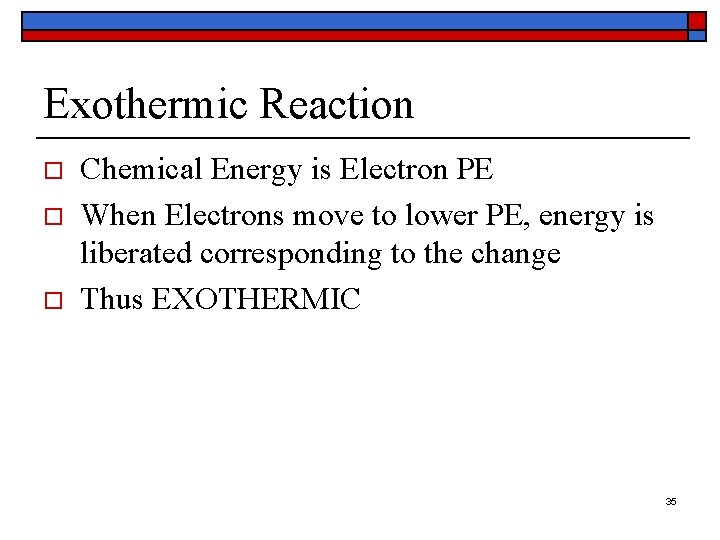Exothermic Reaction o o o Chemical Energy is Electron PE When Electrons move to lower PE, energy is liberated corresponding to the change Thus EXOTHERMIC 35Reaction Rates o What affects how quickly or slowly (reaction rate) a chemical reaction will occur? n n Temperature – higher temps mean faster reaction rates Concentration – the rate of a simple chemical reaction is proportional to the concentration of each reacting substance Surface Area – the greater the surface area, the more quickly atoms and molecules can get together to react Catalysts – substances that either speed up or slow down a reaction without being permanently changed themselves 36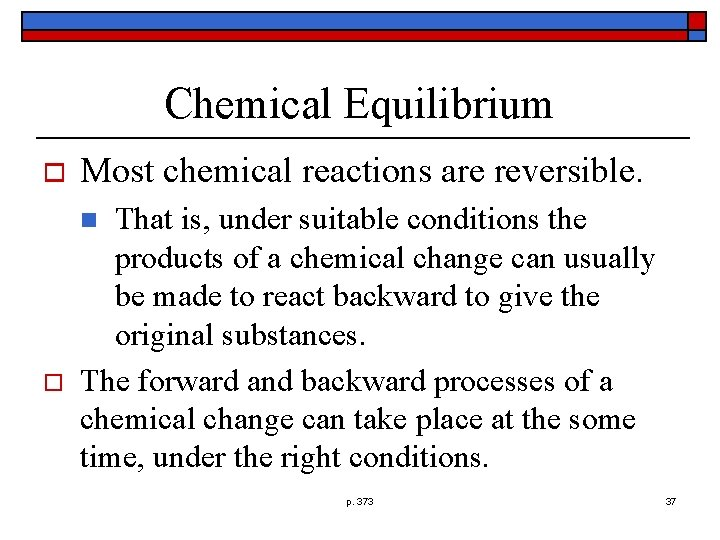Chemical Equilibrium o Most chemical reactions are reversible. That is, under suitable conditions the products of a chemical change can usually be made to react backward to give the original substances. The forward and backward processes of a chemical change can take place at the some time, under the right conditions. n o p. 373 37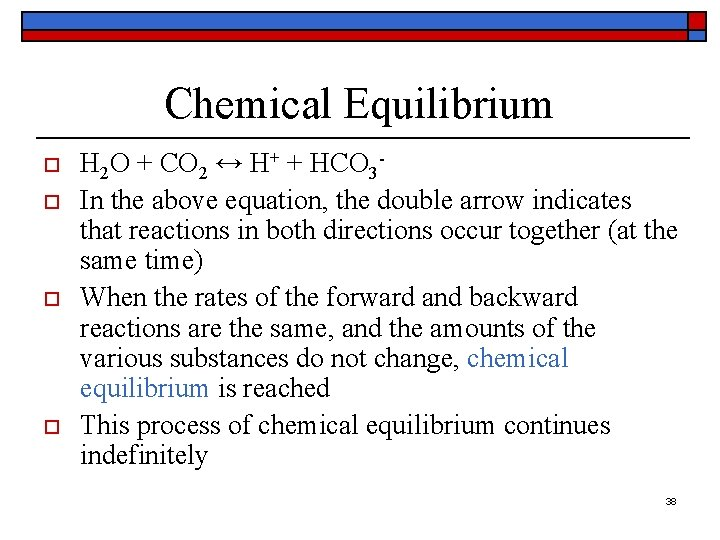Chemical Equilibrium o o H 2 O + CO 2 ↔ H+ + HCO 3 In the above equation, the double arrow indicates that reactions in both directions occur together (at the same time) When the rates of the forward and backward reactions are the same, and the amounts of the various substances do not change, chemical equilibrium is reached This process of chemical equilibrium continues indefinitely 38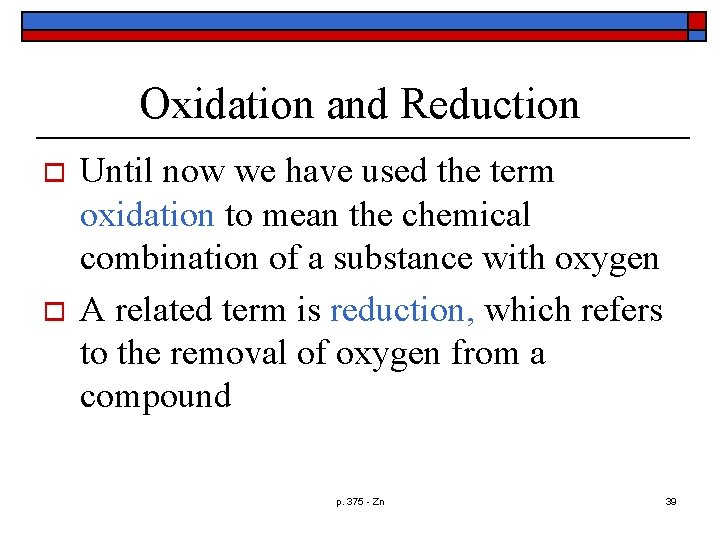Oxidation and Reduction o o Until now we have used the term oxidation to mean the chemical combination of a substance with oxygen A related term is reduction, which refers to the removal of oxygen from a compound p. 375 - Zn 39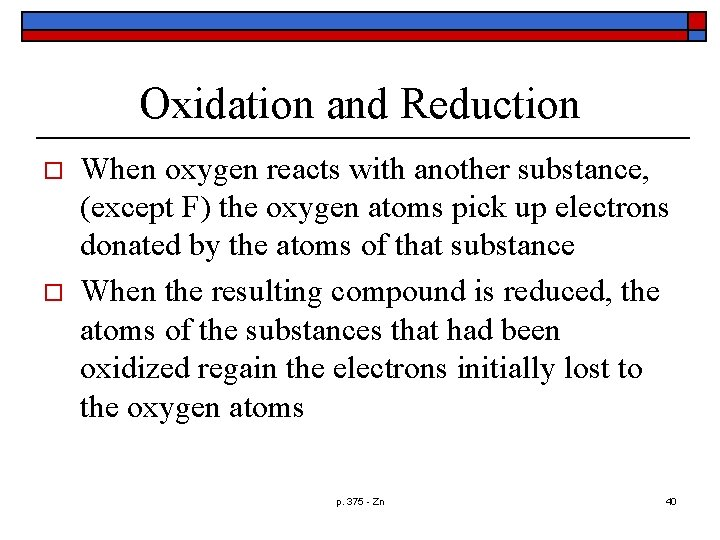Oxidation and Reduction o o When oxygen reacts with another substance, (except F) the oxygen atoms pick up electrons donated by the atoms of that substance When the resulting compound is reduced, the atoms of the substances that had been oxidized regain the electrons initially lost to the oxygen atoms p. 375 - Zn 40Oxidation and Reduction o o Oxidation refers to the loss of electrons by the atoms of an element Reduction refers to the gain of electrons by the atoms of an element The oxidation of one element is always accompanied by the reduction of another The two processes must take place together p. 375 Cl 41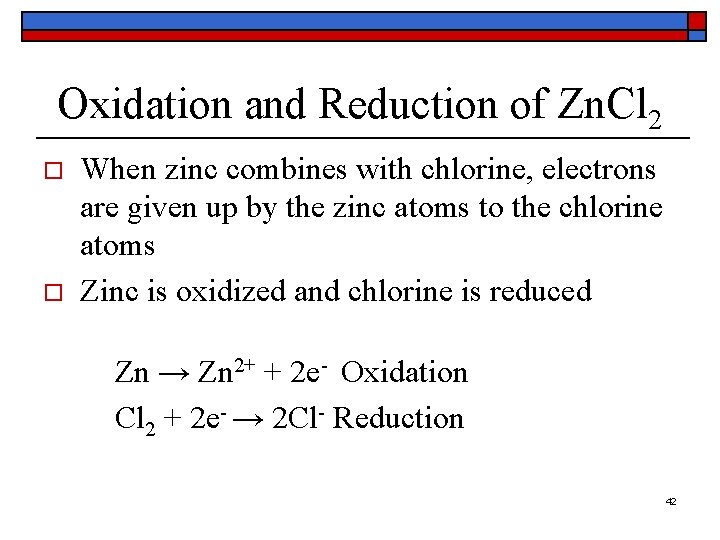Oxidation and Reduction of Zn. Cl 2 o o When zinc combines with chlorine, electrons are given up by the zinc atoms to the chlorine atoms Zinc is oxidized and chlorine is reduced Zn → Zn 2+ + 2 e- Oxidation Cl 2 + 2 e- → 2 Cl- Reduction 42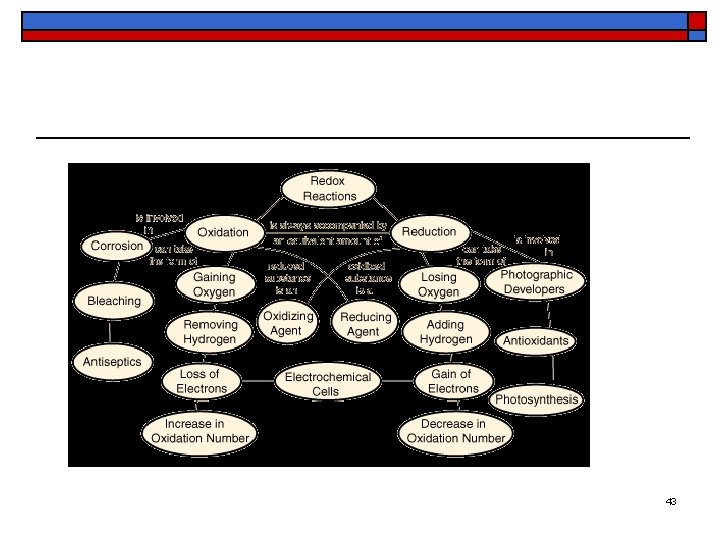43o http: //www. metacafe. com/watch/yt_Pk 6 s 1 Mbsz. A/gummy_bear_oxidation/ 44